# NCERT Solutions for Class 10 Maths Exercise 5.1## myCBSEguide App

CBSE, NCERT, JEE Main, NEET-UG, NDA, Exam Papers, Question Bank, NCERT Solutions, Exemplars, Revision Notes, Free Videos, MCQ Tests & more.

NCERT solutions for Maths Arithmetic Progression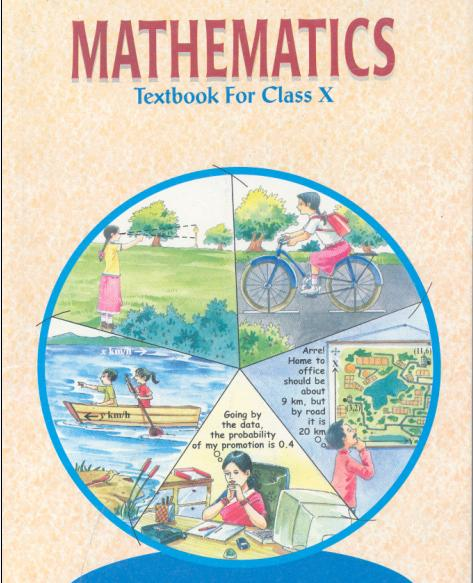## NCERT Solutions for Class 10 Maths Arithmetic Progressions

###### 1. In which of the following situations, does the list of numbers involved make an arithmetic progression, and why?

(i) The taxi fare after each km when the fare is Rs 15 for the first km and Rs 8 for each additional km.

(ii) The amount of air present in a cylinder when a vacuum pump removes 14th of the air remaining in the cylinder at a time.

(iii) The cost of digging a well after every meter of digging, when it costs Rs 150 for the first meter and rises by Rs 50 for each subsequent meter.

(iv) The amount of money in the account every year, when Rs 10,000 is deposited at compound Interest at 8% per annum.

Ans. (i)Taxi fare for 1st km = Rs 15, Taxi fare after 2 km = 15 + 8 = Rs 23

Taxi fare after 3 km = 23 + 8 = Rs 31

Taxi fare after 4 km = 31 + 8 = Rs 39

Therefore, the sequence is 15, 23, 31, 39…

It is an arithmetic progression because difference between any two consecutive terms is equal which is 8. (23 – 15 = 8, 31 – 23 = 8, 39 – 31 = 8, …)

(ii)Let amount of air initially present in a cylinder = V

Amount of air left after pumping out air by vacuum pump =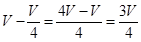Amount of air left when vacuum pump again pumps out air

=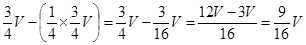So, the sequence we get is like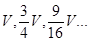Checking for difference between consecutive terms …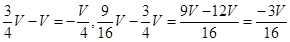Difference between consecutive terms is not equal.

Therefore, it is not an arithmetic progression.

(iii) Cost of digging 1 meter of well = Rs 150

Cost of digging 2 meters of well = 150 + 50 = Rs 200

Cost of digging 3 meters of well = 200 + 50 = Rs 250

Therefore, we get a sequence of the form 150, 200, 250…

It is an arithmetic progression because difference between any two consecutive terms is equal. (200 – 150 = 250 – 200 = 50…)

Here, difference between any two consecutive terms which is also called common difference is equal to 50.

(iv)Amount in bank after Ist year =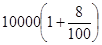… (1)

Amount in bank after two years =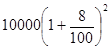… (2)

Amount in bank after three years =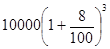… (3)

Amount in bank after four years =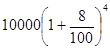… (4)

It is not an arithmetic progression because (2) − (1) ≠ (3) − (2)

(Difference between consecutive terms is not equal)

Therefore, it is not an Arithmetic Progression.

NCERT Solutions for Class 10 Maths Exercise 5.1

###### 2. Write first four terms of the AP, when the first term a and common difference d are given as follows:

(i)a = 10, d = 10

(ii) a = -2, d = 0

(iii) a = 4, d = -3

(iv) a = -1, d =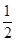(v) a = -1.25, d = -0.25

Ans. (i) First term = a = 10, d = 10

Second term = a + d = 10 + 10 = 20

Third term = second term + d = 20 + 10 = 30

Fourth term = third term + d = 30 + 10 = 40

Therefore, first four terms are: 10, 20, 30, 40

(ii) First term = a = –2 , d = 0

Second term = a + d = –2 + 0 = –2

Third term = second term + d = –2 + 0 = –2

Fourth term = third term + d = –2 + 0 = –2

Therefore, first four terms are: –2, –2, –2, –2

(iii) First term = a = 4, d =–3

Second term = a + d = 4 – 3 = 1

Third term = second term + d = 1 – 3 = –2

Fourth term = third term + d = –2 – 3 = –5

Therefore, first four terms are: 4, 1, –2, –5

(iv) First term = a = –1, d =Second term = a + d = –1 += −Third term = second term + d = −+= 0

Fourth term = third term + d = 0 +=Therefore, first four terms are: –1, −, 0,(v) First term = a = –1.25, d = –0.25

Second term = a + d = –1.25 – 0.25 = –1.50

Third term = second term + d = –1.50 – 0.25 = –1.75

Fourth term = third term + d

= –1.75 – 0.25 = –2.00

Therefore, first four terms are: –1.25, –1.50, –1.75, –2.00

NCERT Solutions for Class 10 Maths Exercise 5.1

###### 3. For the following APs, write the first term and the common difference.

(i) 3, 1, –1, –3 …

(ii) –5, –1, 3, 7…

(iii)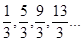(iv) 0.6, 1.7, 2.8, 3.9 …

Ans. (i) 3, 1, –1, –3…

First term = a = 3,

Common difference (d) = Second term – first term = Third term – second term and so on

Therefore, Common difference (d) = 1 – 3 = –2

NCERT Solutions for Class 10 Maths Exercise 5.1

(ii) –5, –1, 3, 7…

First term = a = –5

Common difference (d) = Second term – First term

= Third term – Second term and so on

Therefore, Common difference (d) = –1 – (–5) = –1 + 5 = 4

(iii)First term = a =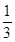Common difference (d) = Second term – First term

= Third term – Second term and so on

Therefore, Common difference (d) =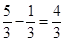(iv) 0.6, 1.7, 2.8, 3.9…

First term = a = 0.6

Common difference (d) = Second term – First term

= Third term – Second term and so on

Therefore, Common difference (d) = 1.7 − 0.6 = 1.1

NCERT Solutions for Class 10 Maths Exercise 5.1

###### 4. Which of the following are APs? If they form an AP, find the common difference d and write three more terms.

(i) 2, 4, 8, 16…

(ii) 2,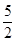, 3,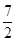(iii) −1.2, −3.2, −5.2, −7.2…

(iv) −10, −6, −2, 2…

(v)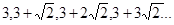(vi) 0.2, 0.22, 0.222, 0.2222…

(vii) 0, −4, −8, −12…

(viii)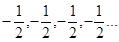(ix) 1, 3, 9, 27…

(x) a, 2a, 3a, 4a

(xi)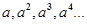(xii)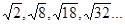(xiii)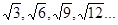(xiv)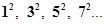(xv)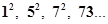Ans. (i) 2, 4, 8, 16…

It is not an AP because difference between consecutive terms is not equal.

As4 – 2 ≠ 8 − 4

(ii)2,, 3,It is an AP because difference between consecutive terms is equal.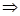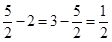Common difference (d) =Fifth term =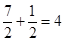Sixth term = 4 + ½ =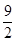Seventh term =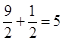Therefore, next three terms are 4,and 5.

(iii)−1.2, −3.2, −5.2, −7.2…

It is an AP because difference between consecutive terms is equal.−3.2 − (−1.2)

= −5.2 − (−3.2)

= −7.2 − (−5.2) = −2

Common difference (d) = −2

Fifth term = −7.2 – 2 = −9.2Sixth term = −9.2 – 2 = −11.2

Seventh term = −11.2 – 2 = −13.2

Therefore, next three terms are −9.2, −11.2 and −13.2

(iv) −10, −6, −2, 2…

It isan AP because difference between consecutive terms is equal.−6 − (−10) = −2 − (−6)

= 2 − (−2) = 4

Common difference (d) = 4

Fifth term = 2 + 4 = 6 Sixth term = 6 + 4 = 10

Seventh term = 10 + 4 = 14

Therefore, next three terms are 6, 10 and 14

NCERT Solutions for Class 10 Maths Exercise 5.1

(v)It is an AP because difference between consecutive terms is equal.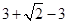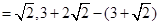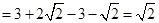Common difference (d) =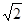Fifth term =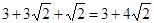Sixth term =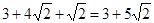Seventh term =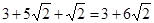Therefore, next three terms are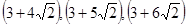(vi) 0.2, 0.22, 0.222, 0.2222…

It is not an AP because difference between consecutive terms is not equal.0.22 − 0.2 ≠ 0.222 − 0.22

(vii) 0, −4, −8, −12…

It is an AP because difference between consecutive terms is equal.−4 – 0 = −8 − (−4)

= −12 − (−8) = −4

Common difference (d) = −4

Fifth term = −12 – 4 =−16 Sixth term = −16 – 4 = −20

Seventh term = −20 – 4 = −24

Therefore, next three terms are −16, −20 and −24

NCERT Solutions for Class 10 Maths Exercise 5.1

(viii)It is an AP because difference between consecutive terms is equal.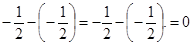Common difference (d) = 0

Fifth term =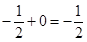Sixth term =Seventh term =Therefore, next three terms are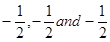(ix) 1, 3, 9, 27…

It is not an AP because difference between consecutive terms is not equal.3 – 1 ≠ 9 − 3

(x) a, 2a, 3a, 4a

It is an AP because difference between consecutive terms is equal.2a a = 3a − 2a = 4a − 3a = a

Common difference (d) = a

Fifth term = 4a + a = 5a Sixth term = 5a + a = 6a

Seventh term = 6a + a = 7a

Therefore, next three terms are 5a, 6a and 7a

(xi) a, a2, a3, a4

It is not an AP because difference between consecutive terms is not equal.a2a a3a2

(xii)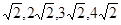It is an AP because difference between consecutive terms is equal.

NCERT Solutions for Class 10 Maths Exercise 5.1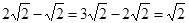Common difference (d) =Fifth term =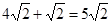Sixth term =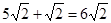Seventh term =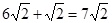Therefore, next three terms are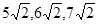(xiii)It is not an AP because difference between consecutive terms is not equal.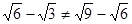(xiv)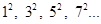It is not an AP because difference between consecutive terms is not equal.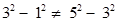(xv)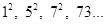1, 25, 49, 73…

It is an AP because difference between consecutive terms is equal.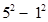=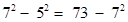= 24

Common difference (d) = 24

Fifth term = 73 + 24 = 97 Sixth term = 97 + 24 = 121

Seventh term = 121 + 24 = 145

Therefore, next three terms are 97, 121 and 145

## NCERT Solutions for Class 10 Maths Exercise 5.1

NCERT Solutions Class 10 Maths PDF (Download) Free from myCBSEguide app and myCBSEguide website. Ncert solution class 10 Maths includes text book solutions from Mathematics Book. NCERT Solutions for CBSE Class 10 Maths have total 15 chapters. 10 Maths NCERT Solutions in PDF for free Download on our website. Ncert Maths class 10 solutions PDF and Maths ncert class 10 PDF solutions with latest modifications and as per the latest CBSE syllabus are only available in myCBSEguide.

## CBSE app for Class 10

To download NCERT Solutions for Class 10 Maths, Computer Science, Home Science,Hindi ,English, Social Science do check myCBSEguide app or website. myCBSEguide provides sample papers with solution, test papers for chapter-wise practice, NCERT solutions, NCERT Exemplar solutions, quick revision notes for ready reference, CBSE guess papers and CBSE important question papers. Sample Paper all are made available through the best app for CBSE students and myCBSEguide website.### 8 thoughts on “NCERT Solutions for Class 10 Maths Exercise 5.1”

1. Good

2. Thank u so much

3. very good

4. Sir thanks for providing questions

5. Thanks it helps me alot

6. Nice I got so many problems

7. You are the best

8. Thank you